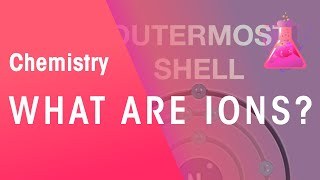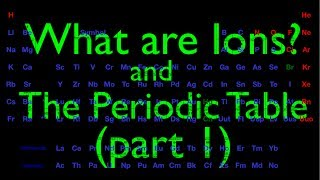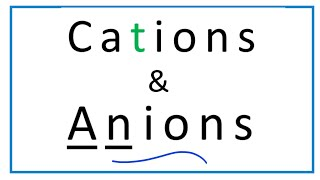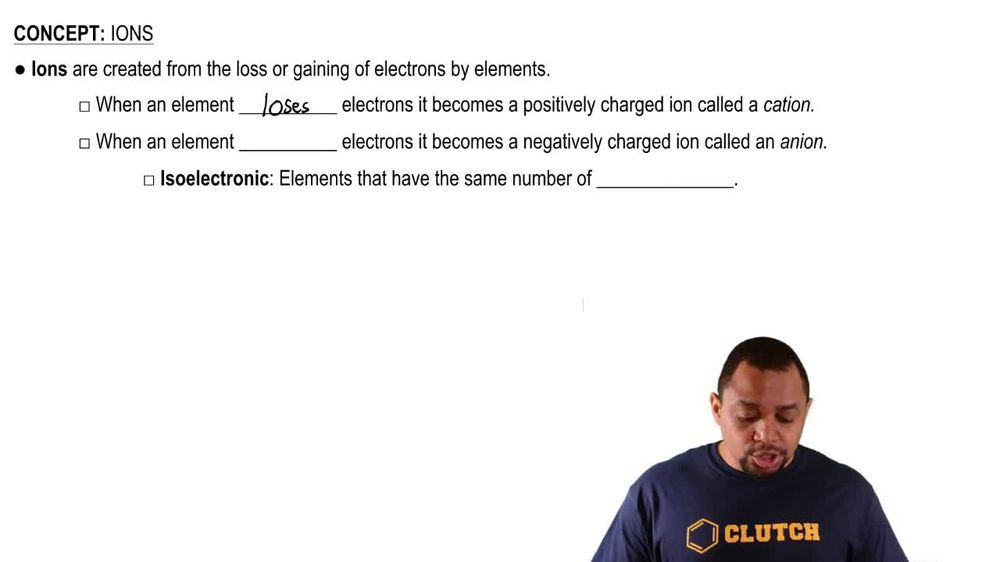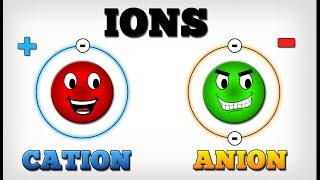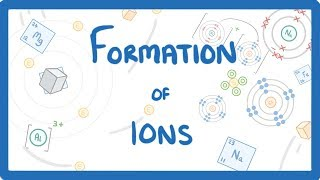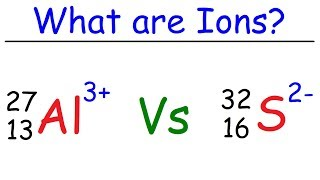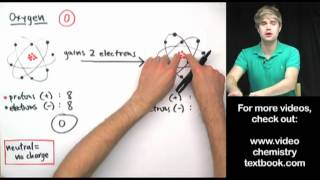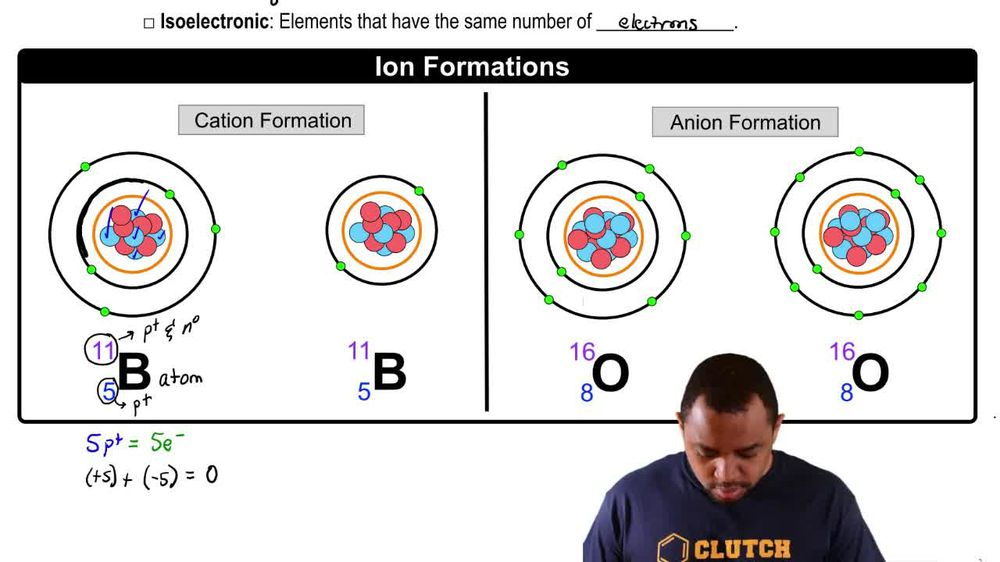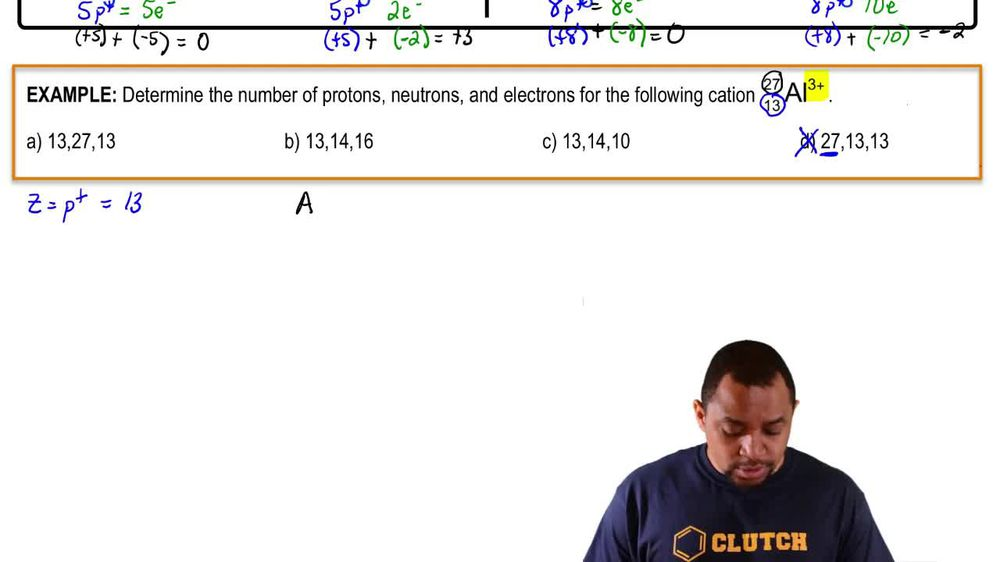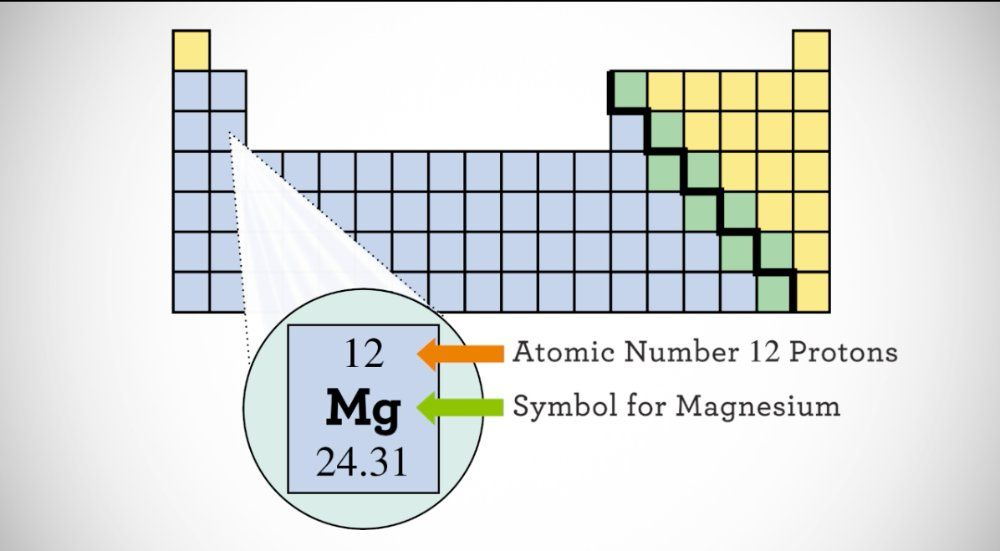Start typing, then use the up and down arrows to select an option from the list.
1. 2. Atoms & Elements2. Ions
Problem

# Fill in the gaps in the following table, assuming each column represents a neutral atom. Symbol 159Tb Protons 29 37 Neutrons 34 53 Electrons 42 34 Mass no. 79 85 Complete the fourth row of the table.

Relevant Solution3m
Play a video:
everyone in this example, we need to fill in the fourth row of our given table. So the fourth row is going to correspond to the section describing electrons. And in the first column of the fourth row, we notice we're given the F E three plus carry on and the mass number 58. So writing out that chemical symbol, we would have F E three plus. And recall that the mass number is always written in the left hand superscript of our chemical symbol. We want to recall that our mass number is found from taking our number of protons and adding it to our number of neutrons. And so we want to find our number of electrons. So we're going to focus on finding the number of protons first by considering a neutral atom of iron Where we find on our periodic tables. Iron in the transition middle section corresponding to atomic number 26. And we want to recall that the atomic number is always written in the left hand subscript of our chemical symbol for a given atom. And so we want to recall that the atomic number will correspond to our number of protons, which will equal our number of electrons in a neutral atom. But in this case we don't have a neutral atom. We have a three plus catalon, meaning that we will lose Three electrons. And so we want to recall that because we have 26 electrons in a neutral atom of iron based on our number of protons, in our atomic number For a charged atom of iron with a three plus charge. We would subtract three electrons leaving us with 23 electrons to fill in for our first column of our fourth row. So we have 23 electrons. And then for the third row of our fourth column we are given the number of protons, the number of neutrons and a net charge. So again, we want to find based on our number of protons we want to recall that corresponds to our atomic number. So we want to find atomic number 76 on the periodic table because we're given 76 protons and to find 76 on the periodic table, we would see that that corresponds to the atom osmium or Os. And so we want to recall again that the atomic number is written in the left hand subscript of our chemical symbol. And so to find our number of electrons, we want to recognize that we have a net charge of plus one on this atom. So we would fill that in on the right hand side and recall that the plus one charge means we lose one electron. And so assuming that in a neutral atom of osmium We would have 76 protons and 76 electrons For a charged atom of osmium with a plus one charge. We would take those 76 electrons and we would subtract one electron leaving us with 75 electrons. And so we would say that to fill in the third column of the 4th row. We would have 75 electrons as our answer here. And so to complete this example, we would have these two boxed in yellow, um, answers as our final answer. So I hope that everything we reviewed is clear. If you have any questions, please leave them down below, and I will see everyone in the next practice video.